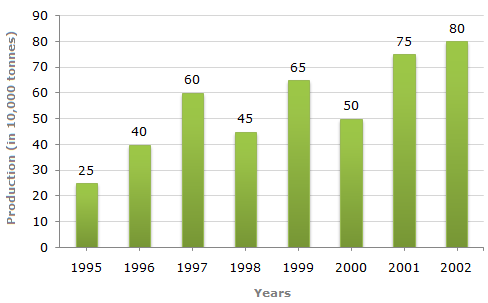# Data Interpretation - Bar Charts

Directions to Solve

Study the bar chart and answer the question based on it.

Production of Fertilizers by a Company (in 1000 tonnes) Over the Years1.
What was the percentage decline in the production of fertilizers from 1997 to 1998?
33(1/3)%
20%
25%
21%
Explanation:

 Required percentage =(45 - 60)% = -25%. 60There is a decline of 25% in production from 1997 to 1998.

2.
The average production of 1996 and 1997 was exactly equal to the average production of which of the following pairs of years?
2000 and 2001
1999 and 2000
1998 and 2000
1995 and 2001
Explanation:

 Average production (in 10000 tonnes) of 1996 and 1997 40 + 60 = 50. 2

We shall find the average production (in 10000 tonnes) for each of the given alternative pairs:

 2000 and 2001 = 50 + 75 = 62.5. 2

 1999 and 2000 = 65 + 50 = 57.5. 2

 1998 and 2000 = 45 + 50 = 47.5. 2

 1995 and 1999 = 25 + 65 = 45. 2

 1995 and 2001 = 25 + 75 = 50. 2The average production of 1996 and 1997 is equal to the average production of 1995 and 2001.

3.
What was the percentage increase in production of fertilizers in 2002 compared to that in 1995?
320%
300%
220%
200%
Explanation:

 Required percentage =(80 - 25) x 100% = 220%. 25

4.
In which year was the percentage increase in production as compared to the previous year the maximum?
2002
2001
1997
1996
Explanation:

The percentage increase in production compared to previous year for different years are:

 In 1996 =(40 - 25) x 100% = 60%. 25

 In 1997 =(60 - 40) x 100% = 50%. 40

In 1998 there is a decrease in production.

 In 1999 =(65 - 45) x 100% = 44.44%. 45

In 2000 there is a decrease in production.

 In 2001 =(75 - 50) x 100% = 50%. 50

 In 2002 =(80 - 75) x 100% = 6.67%. 75

Clearlyl, there is maximum percentage increase in production in 1996.

5.
In how many of the given years was the production of fertilizers more than the average production of the given years?
1
2
3
4The productions during the years 1997, 1999, 2001 and 2002 are more than the average production.